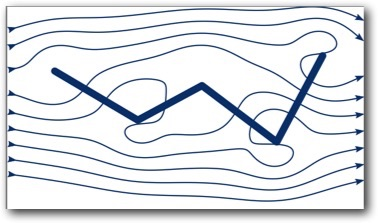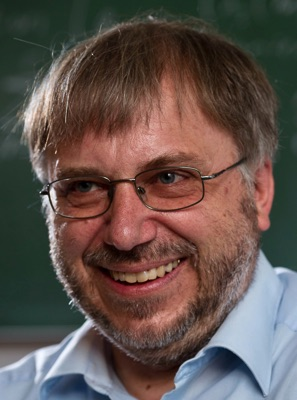Christian Klingenberg

research interests:

Mathematical Fluid Mechanics

theory of hyperbolic conservation laws

•   towards a proper solution concept for the multi-dim. compressible Euler equations

•   convex integration, non-uniqueness for the multi-dim. compressible Euler equations

structure preserving numerical schemes for Euler and ideal magnetohydrodynamics

•   well-balanced and low Mach schemes for Euler equations with gravity

•   genuinely multi-dim. schemes

•   structure-preserving compact high-order method method

applications in astrophysics

•   numerical schemes for evolving stellar structure and evolution

•   simulations of the evolution of the universe including magnetic fields

kinetic equations and plasma, theory & numerics

•   multi-species models, existence and qualitative behavior

•   numerical schemes for multi-species BGK equations based on a variational procedure

PDE inverse problems, kinetic models in biology with coefficients determined by experimental data

•   theory: proving that it is possible to solve certain kinetic PDE inverse problems

•   numerics: schemes that determine the coefficients from given experimental dataOur logo, showing the interplay between astrophysics (Cassiopeia) and fluid mechanics.

designed together with Sebastian Ohlmann

former workshops, conferences etc.:

- Seminar series from Sept. 2020 - May 2022 Hyperbolic equations: Structure preserving numerical methods and other topics, see here

- Oberwolfach workshop "Structure preserving numerical methods for hyperbolic equations", April 11 - 15, 2022 see here

- Oberwolfach workshop "Modelling phenomena from nature by hyperbolci conservation laws", April 11, 17, 2021 see here

- Fall school Oct. 1 - 5, 2018 in Würzburg: Hyperbolic conservation laws and mathematics of fluid mechanics, with lectures by   Constantine Dafermos, Eduard Feireisl, Sara Daneri and Simon Markfelder

- 16th International Conference on Hyperbolic Problems, organized by M. Westdickenberg and myself Aug. 1 - 5, 2016.

- Workshop in Würzburg, June 30 - July 3, 2015,Evolutionary Equations, theory & numerics”, see here

Dept. of Mathematics

Würzburg University

Emil Fischer Str. 40

97074 Würzburg

Germany

I am in room 03.011 (top floor)

phone: (0049) (0)931 318 5045

email:    klingen "at" mathematik.uni-wuerzburg.de

upcoming visitors:

- Yunguang Lu (Hangzhou Normal University, China), June 3 - Aug. 1, 2023

- Maria Han-Veiga (Univ. of Michigan. Ann Arbor, USA), June 11 - 16, 2023

- Emil Wiedemann (Univ. Erlangen), July 11, 2023